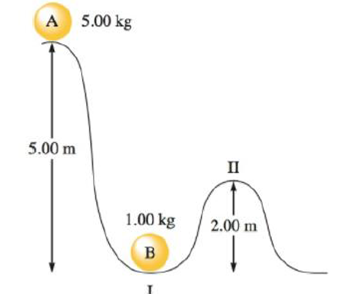# Consider the accompanying diagram. Ball A is allowed to fall and strike ball B. Assume that all of ball A’s energy is transferred to ball B at point I, and that there is no loss of energy to other sources. What is the kinetic energy and the potential energy of ball B at point II? The potential energy is given by PE = mgz, where m is the mass in kilograms, g is the gravitational constant (9.81 m/s 2 ), and z is the distance in meters.### Chemistry: An Atoms First Approach

2nd Edition
Steven S. Zumdahl + 1 other
Publisher: Cengage Learning
ISBN: 9781305079243

#### Solutions

Chapter
Section### Chemistry: An Atoms First Approach

2nd Edition
Steven S. Zumdahl + 1 other
Publisher: Cengage Learning
ISBN: 9781305079243
Chapter 7, Problem 28E
Textbook Problem
231 views

## Consider the accompanying diagram. Ball A is allowed to fall and strike ball B. Assume that all of ball A’s energy is transferred to ball B at point I, and that there is no loss of energy to other sources. What is the kinetic energy and the potential energy of ball B at point II? The potential energy is given by PE = mgz, where m is the mass in kilograms, g is the gravitational constant (9.81 m/s2), and z is the distance in meters.Interpretation Introduction

Interpretation: The kinetic energy and potential energy of ball B at point II should be calculated.

Concept Introduction:

• Potential energy is the energy of an object result of its position. For the gravitational potential energy has,

Formula:    PEgrav = mass × g × height......(1)

• Kinetic energy is an energy of an object due to the motion of it.

Formula:

KE=12mv2......(2)

m is mass of object (g).

v is velocity of object (m/s)

### Explanation of Solution

Explanation

From the given data,

Mass of ball A at the point is 2.00 kg

Mass of ball B at point II is 4.00 kg

Gravitational constant 9.81 m/s2

Velocity of ball A at the point is 10.0 m

Velocity of ball B at the point II is 3.00m

The calculated mass and velocity of the balls A, B are and gravitational constant values are recorded as shown above.

The potential energy of ball A is calculated as,

PEgrav = mass × g × height......(1)

PEballA=2.00kg×9.81ms2×10.0m=196kgm2s2=196J

The given values are substitute in equation (1) to get the potential energy of ball A is 196J

### Still sussing out bartleby?

Check out a sample textbook solution.

See a sample solution

#### The Solution to Your Study Problems

Bartleby provides explanations to thousands of textbook problems written by our experts, many with advanced degrees!

Get Started